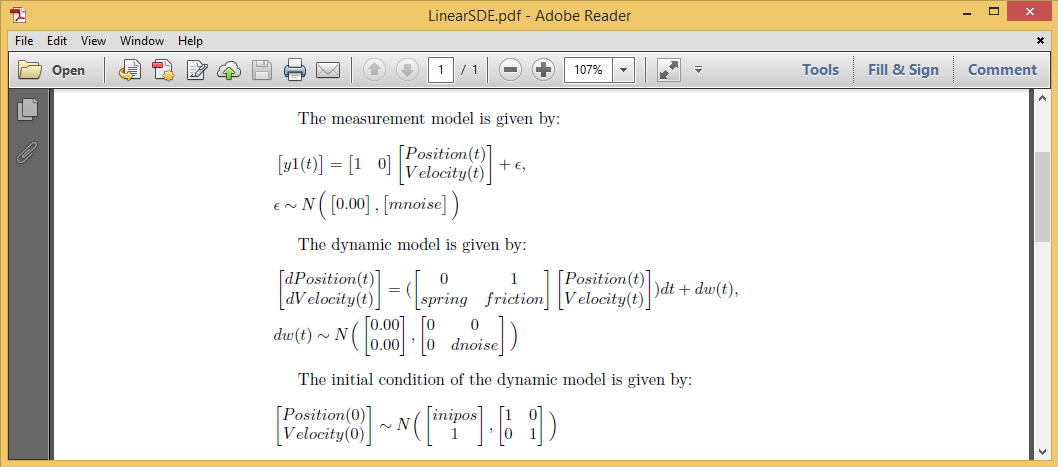# Example: A Linear Stochastic Differential Equation Model

## Introduction

This example illustrates how to fit a linear Stochastic differential equation (SDE) model in the dynr package.

A damped linear oscillator model is estimated. Details of the model set up is as follows:

$$\frac{d^2x}{dt^2} = -kx - c\frac{dx}{dt} + \zeta$$

This model can also be written in the form of a vector of first-order systems:

The dynamic model is :

$\begin{pmatrix} \frac{dx}{dt}\\ \frac{d^2x}{dt^2} \end{pmatrix} = \begin{pmatrix} 0 & 1\\ -k & -c \end{pmatrix}\begin{pmatrix} x\\ \frac{dx}{dt} \end{pmatrix} + \begin{pmatrix} 0\\ \zeta \end{pmatrix}$

The measurement model is:

$y = \begin{pmatrix} 1 & 0 \end{pmatrix}\begin{pmatrix} x\\ \frac{dx}{dt} \end{pmatrix} + \epsilon$

## Data

The dynr.data command is used to create a dynr data object. Here the observed variable we are modeling is “y1” . (The simulated data are loaded as part of the package.)

require(dynr)
## Loading required package: dynr
## Loading required package: ggplot2
# Data
data(Oscillator)
data <- dynr.data(Oscillator, id="id", time="times", observed="y1")

## Measurement Model

The prep.measurement command is used to specify the factor loadings matrix. The values.load argument specifies that the factor loadings are fixed at 1 and 0. In this model, all parameters are fixed, which is indicated by the params.load argument. The *.names argument gives names to the latent and observed variables.

meas <- prep.measurement(
state.names=c("Position","Velocity"),
obs.names=c("y1"))

## Dynamic Model

The prep.noise command is used to specify dynamic (values.latent) and observation (values.observed) noise components. The latent noise is the dynamic noise. The observed noise is the measurement noise.

The prep.matrixDynamics command is used to define the differental equation. In this model, the first row of the parameter matrix is fixed at 0 and 1 and the starting values for the second row of the matrix is set at -0.1 and -0.2.

ecov <- prep.noise(
values.latent=diag(c(0, 1), 2), params.latent=diag(c('fixed', 'dnoise'), 2),
values.observed=diag(1.5, 1), params.observed=diag('mnoise', 1))
dynamics <- prep.matrixDynamics(
values.dyn=matrix(c(0, -0.1, 1, -0.2), 2, 2),
params.dyn=matrix(c('fixed', 'spring', 'fixed', 'friction'), 2, 2),
isContinuousTime=TRUE)

## Initial Values

This step specifies the covariances and latent state values at t=0. These initialize the recursive algorithm (extended Kalman filter) that dynr uses.

initial <- prep.initial(
values.inistate=c(0, 1),
params.inistate=c('inipos', 'fixed'),
values.inicov=diag(1, 2),
params.inicov=diag('fixed', 2)) 

## Dynr Model

Now we put together everything we’ve previously specified in dynr.model. This code connects the recipes we’ve written up with our data and writes a c file in our working directory. We can inspect c functions that go with each recipe in the c file.

model <- dynr.model(dynamics=dynamics, measurement=meas, noise=ecov, initial=initial, data=data, outfile="LinearSDE.c")

## Tex Options

We can check our model specifications in a neatly printed pdf file using the following code.

The printex command is used to write the model into a Latex file, with a name given by the outFile argument. Then, the tools::texi2pdf command generates a pdf file from the latex file we just created. The system command prints out the pdf file:We can also print out the model in R, instead of generating a Latex file, using the command plotFormula.

printex(model,ParameterAs=model\$param.names,show=FALSE,printInit=TRUE,
outFile="LinearSDE.tex")
tools::texi2pdf("LinearSDE.tex")
system(paste(getOption("pdfviewer"), "LinearSDE.pdf"))

## Optimization Step and Results

Now the model is specified and we are ready to cook dynr! The summary command gives the model fitting results.

res <- dynr.cook(model, verbose=FALSE)
summary(res)